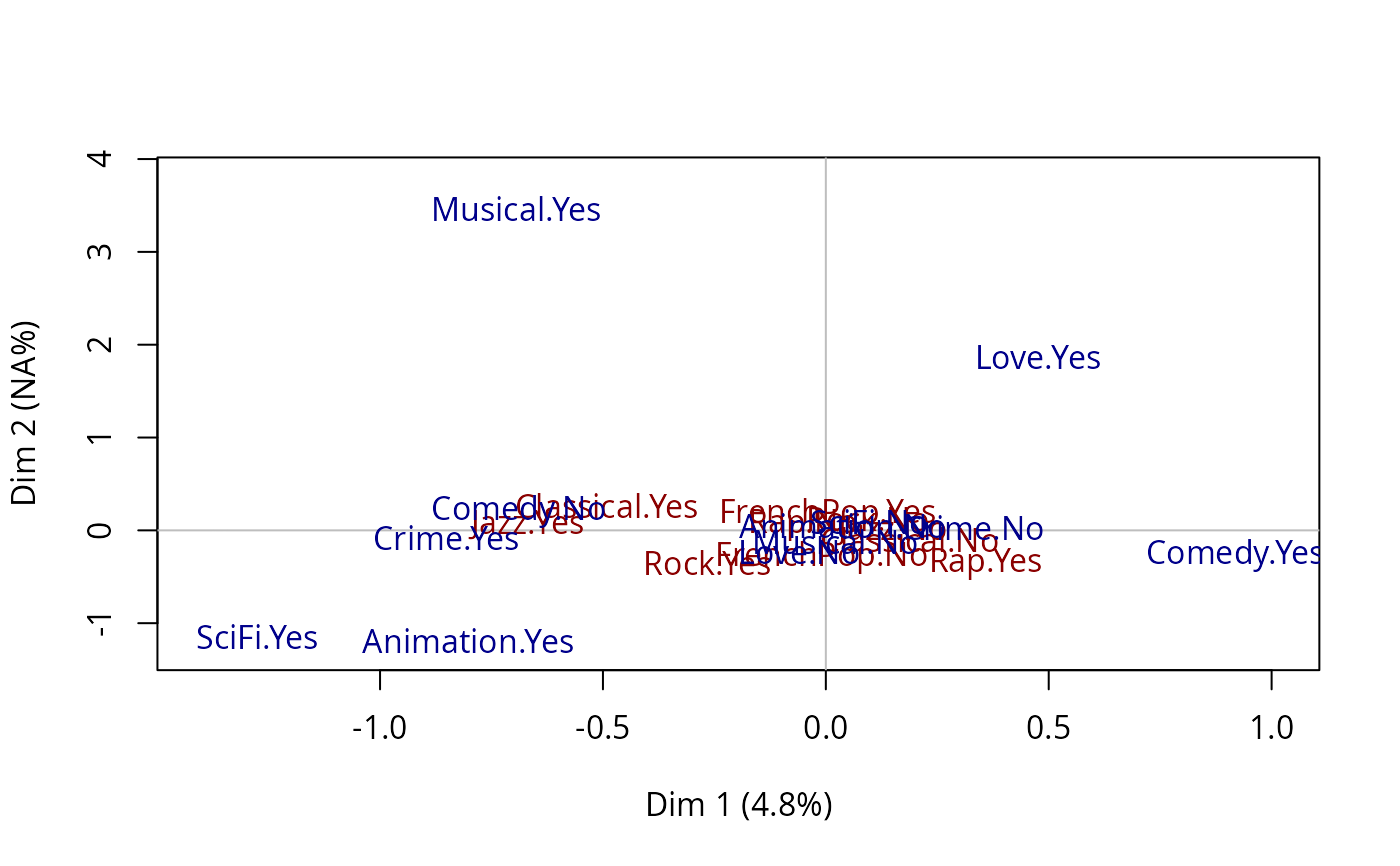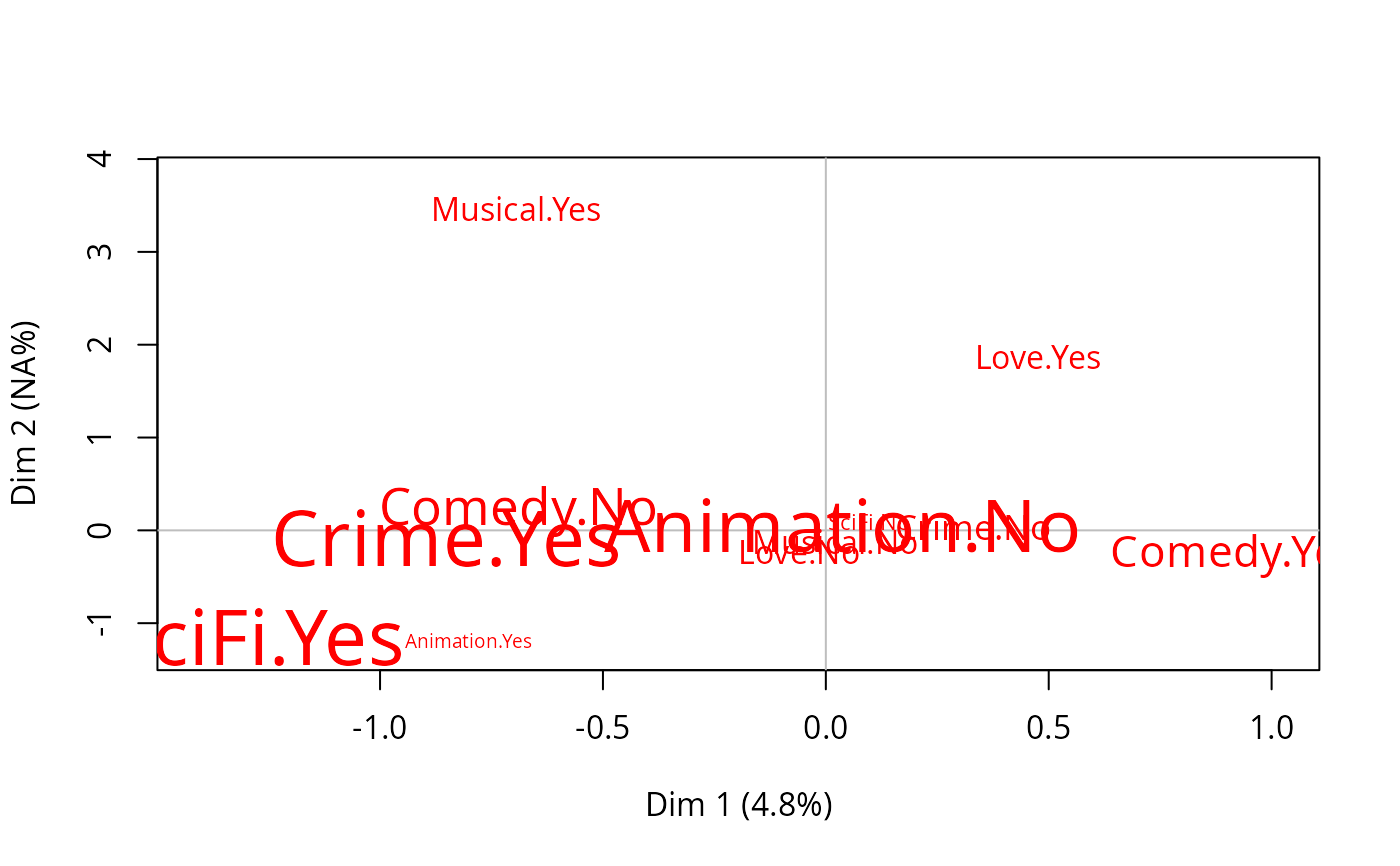Plots Multiple Factor Analysis data, resulting from multiMCA function.

# S3 method for multiMCA
plot(x, type = "v", axes = c(1, 2), points = "all", threshold = 2.58,
groups = 1:x$call$ngroups, col = rainbow(x$call$ngroups), app = 0, ...)

## Arguments

x

object of class multiMCA

type

character string: 'v' to plot the categories (default), 'i' to plot individuals' points, 'inames' to plot individuals' names

axes

numeric vector of length 2, specifying the components (axes) to plot (c(1,2) is default)

points

character string. If 'all' all points are plotted (default); if 'besth' only those who are the most correlated to horizontal axis are plotted; if 'bestv' only those who are the most correlated to vertical axis are plotted; if 'best' only those who are the most coorelated to horizontal or vertical axis are plotted.

threshold

numeric value. V-test minimal value for the selection of plotted categories.

groups

numeric vector specifying the groups of categories to plot. By default, every groups of categories will be plotted

col

a color for the points of the individuals or a vector of colors for the labels of the groups of categories (by default, rainbow palette is used)

app

numerical value. If 0 (default), only the labels of the categories are plotted and their size is constant; if 1, only the labels are plotted and their size is proportional to the weights of the categories; if 2, points (triangles) and labels are plotted, and points size is proportional to the weight of the categories.

...

further arguments passed to or from other methods, such as cex, cex.main, ...

## Details

A category is considered to be one of the most correlated to a given axis if its test-value is higher then 2.58 (which corresponds to a 0.05 threshold).

## Author

Nicolas Robette

multiMCA, textvarsup, speMCA, csMCA

## Examples

# specific MCA on music variables of Taste example data set
## another one on movie variables of 'Taste' example data set,
## and then a Multiple Factor Analysis and plots the results.
data(Taste)
# specific MCA on music variables of Taste example data set
mca1 <- speMCA(Taste[,1:5], excl = c(3,6,9,12,15))
# specific MCA on movie variables of Taste example data set
mca2 <- speMCA(Taste[,6:11], excl = c(3,6,9,12,15,18))
# Multiple Factor Analysis
mfa <- multiMCA(list(mca1,mca2))
# plot
plot.multiMCA(mfa, col = c("darkred", "darkblue"))# plot of the second set of variables (movie)
plot.multiMCA(mfa, groups = 2, app = 1)